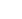# How many tissues should the Kimberly Clark Corporation package of Kleenex contain? Researchers determined that 60 tissues is the mean number of tissues used during a cold.

How many tissues should the Kimberly Clark Corporation package of Kleenex contain? Researchers determined that 60 tissues is the mean number of tissues used during a cold. Suppose a random sample of 100 Kleenex users yielded the following data on the number of tissues used during a cold: = 52, S = 22. Give the null and alternative hypotheses to determine if the number of tissues used during a cold is less than 60. a.) H0: ? ? 60 and H1: ? > 60. b.) H0: ? ? 60 and H1: ? < 60. c.) H0: ? 60 and H1: < 60. d.) H0: = 52 and H1: ? 52. 2.) SCENARIO 9-1 Microsoft Excel was used on a set of data involving the number of defective items found in a random sample of 46 cases of light bulbs produced during a morning shift at a plant. A manager wants to know if the mean number of defective bulbs per case is greater than 20 during the morning shift. She will make her decision using a test with a level of significance of 0.10. The following information was extracted from the Microsoft Excel output for the sample of 46 cases: n = 46; Arithmetic Mean = 28.00; Standard Deviation = 25.92; Standard Error = 3.82; Null Hypothesis: H0 : ? ? 20; ? = 0.10; df = 45; T Test Statistic = 2.09; One-Tail Test Upper Critical Value = 1.3006; p-value = 0.021; Decision = Reject. True or False: Referring to Scenario 9-1, if these data were used to perform a two-tail test, the p-value would be 0.042. True False

Automated page speed optimizations for fast site performance